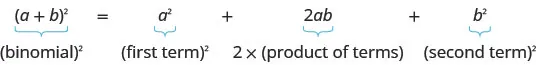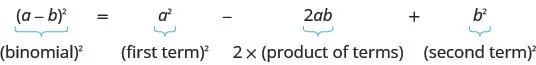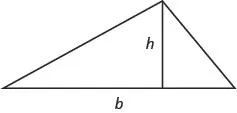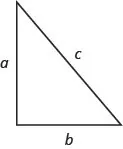Elementary Algebra

# Key Concepts

Elementary AlgebraKey Concepts

### Key Concepts

#### 10.1Solve Quadratic Equations Using the Square Root Property

• Square Root Property
If $x2=kx2=k$, and $k≥0k≥0$, then $x=korx=−kx=korx=−k$.

#### 10.2Solve Quadratic Equations by Completing the Square

• Binomial Squares Pattern If $a,ba,b$ are real numbers,
$(a+b)2=a2+2ab+b2(a+b)2=a2+2ab+b2$$(a−b)2=a2−2ab+b2(a−b)2=a2−2ab+b2$• Complete a Square
To complete the square of $x2+bxx2+bx$:
1. Step 1. Identify $bb$, the coefficient of $xx$.
2. Step 2. Find $(12b)2(12b)2$, the number to complete the square.
3. Step 3. Add the $(12b)2(12b)2$ to $x2+bxx2+bx$.

• Quadratic Formula The solutions to a quadratic equation of the form $ax2+bx+c=0,ax2+bx+c=0,$ $a≠0a≠0$ are given by the formula:
$x=−b±b2−4ac2ax=−b±b2−4ac2a$
1. Step 1. Write the quadratic formula in standard form. Identify the $a,b,ca,b,c$ values.
2. Step 2. Write the quadratic formula. Then substitute in the values of $a,b,c.a,b,c.$
3. Step 3. Simplify.
4. Step 4. Check the solutions.
• Using the Discriminant, $b2−4acb2−4ac$, to Determine the Number of Solutions of a Quadratic Equation
For a quadratic equation of the form $ax2+bx+c=0,ax2+bx+c=0,$ $a≠0,a≠0,$
• if $b2−4ac>0b2−4ac>0$, the equation has 2 solutions.
• if $b2−4ac=0b2−4ac=0$, the equation has 1 solution.
• if $b2−4ac<0b2−4ac<0$, the equation has no real solutions.
• To identify the most appropriate method to solve a quadratic equation:
1. Step 1. Try Factoring first. If the quadratic factors easily this method is very quick.
2. Step 2. Try the Square Root Property next. If the equation fits the form $ax2=kax2=k$ or $a(x−h)2=ka(x−h)2=k$, it can easily be solved by using the Square Root Property.
3. Step 3. Use the Quadratic Formula. Any other quadratic equation is best solved by using the Quadratic Formula.

#### 10.4Solve Applications Modeled by Quadratic Equations

• Area of a Triangle For a triangle with base, $bb$, and height, $hh$, the area, $AA$, is given by the formula: $A=12bhA=12bh$• Pythagorean Theorem In any right triangle, where $aa$ and $bb$ are the lengths of the legs, and $cc$ is the length of the hypothenuse, $a2+b2=c2a2+b2=c2$• Projectile motion The height in feet, $hh$, of an object shot upwards into the air with initial velocity, $v0v0$, after $tt$ seconds can be modeled by the formula:
$h=−16t2+v0th=−16t2+v0t$

• The graph of every quadratic equation is a parabola.
• Parabola Orientation For the quadratic equation $y=ax2+bx+cy=ax2+bx+c$, if
• $a>0a>0$, the parabola opens upward.
• $a<0a<0$, the parabola opens downward.
• Axis of Symmetry and Vertex of a Parabola For a parabola with equation $y=ax2+bx+cy=ax2+bx+c$:
• The axis of symmetry of a parabola is the line $x=−b2ax=−b2a$.
• The vertex is on the axis of symmetry, so its x-coordinate is $−b2a−b2a$.
• To find the y-coordinate of the vertex we substitute $x=−b2ax=−b2a$ into the quadratic equation.
• Find the Intercepts of a Parabola To find the intercepts of a parabola with equation $y=ax2+bx+cy=ax2+bx+c$:
$y-interceptx-interceptsLetx=0and solve fory.Lety=0and solve forx.y-interceptx-interceptsLetx=0and solve fory.Lety=0and solve forx.$
• To Graph a Quadratic Equation in Two Variables
1. Step 1. Write the quadratic equation with $yy$ on one side.
2. Step 2. Determine whether the parabola opens upward or downward.
3. Step 3. Find the axis of symmetry.
4. Step 4. Find the vertex.
5. Step 5. Find the y-intercept. Find the point symmetric to the y-intercept across the axis of symmetry.
6. Step 6. Find the x-intercepts.
7. Step 7. Graph the parabola.
• Minimum or Maximum Values of a Quadratic Equation
• The y-coordinate of the vertex of the graph of a quadratic equation is the
• minimum value of the quadratic equation if the parabola opens upward.
• maximum value of the quadratic equation if the parabola opens downward.
Order a print copy

As an Amazon Associate we earn from qualifying purchases.